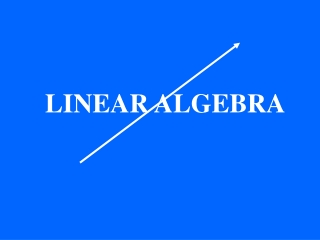DownloadDownload PresentationLINEAR ALGEBRA

# LINEAR ALGEBRA

Télécharger la présentation## LINEAR ALGEBRA

- - - - - - - - - - - - - - - - - - - - - - - - - - - E N D - - - - - - - - - - - - - - - - - - - - - - - - - - -
##### Presentation Transcript

1. LINEARALGEBRA

2. (10 airplanes,15 boats) A toy manufacturer makes airplanes and boats: It costs \$3to make one airplane and \$2to make one boat. He has a total of \$60. The many possibilities can be organized as solutions to 3a + 2b = 60 It takes 2 hoursto make one airplane and 4 hoursto make one boat. He has a total of 80 hoursThe many possibilities can be organized as solutions to 2a + 4b = 80 The system can be solved geometrically - the solution is the intersection of two lines

3. and cars A toy manufacturer makes airplanes and boats It costs \$3to make one airplane , \$2to make one boat and \$1 to make one car. He has a total of \$60. The many possibilities can be organized as solutions to 3a + 2b + 1c = 60 It takes 2 hoursto make one airplane, 4 hoursto make one boat and 6 hoursto make one car. He has a total of 80 hoursThe many possibilities can be organized as solutions to 2a + 4b + 6c = 80

4. A toy manufacturer makes airplanes and boats and cars It costs \$3to make one airplane , \$2to make one boat and \$1 to make one car. He has a total of \$60. The many possibilities can be organized as solutions to 3a + 2b + 1c = 60 It takes 2 hoursto make one airplane, 4 hoursto make one boat and 6 hoursto make one car. He has a total of 80 hoursThe many possibilities can be organized as solutions to 2a + 4b + 6c = 80 The system can be solved geometrically. The solutions are the points at the intersection of two planes

5. In 3 dimensional space, solutions to linear equations and systems can be organized geometrically as lines and points and planes and lines

6. and drums A toy manufacturer makes airplanes and boats, cars It costs \$3to make one airplane , \$2to make one boat ,\$1 to make one car and \$2 to make one drum. He has a total of \$60. Possibilities are solutions to 3a + 2b + 1c + 2d = 60 It takes 2 hoursto make one airplane, 4 hoursto make one boat, 6 hoursto make one car and 1 hourto make one drum. He has a total of 80 hours . Possibilities are solutions to 2a + 4b + 6c + 1d = 80

7. 3a + 2b + 1c + 2d = 60 2a + 4b + 6c + 1d = 80 We are now in four dimensions. It is no longer possible to organize solutions geometrically as points, lines, and planes. We can no longer visualize the solutions. However, the set of solutions has just as much structure as the solutions to the previous problems in two and three dimensions. We must rely on algebra to explore this structure.

8. (2,4) and (-1,-2) (1,2) An example of algebraic structure: The points on the line 2x - y = 0 have an obvious geometric structure and a not so obvious algebraic structure. Choose any two points on the line The sum of these points is also a point on the line We say that this set of points has closure